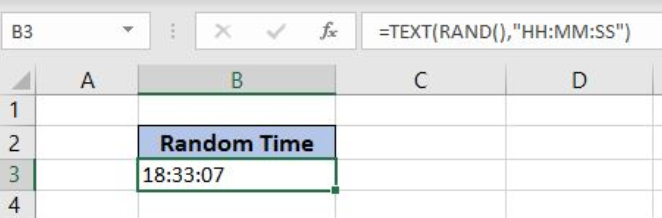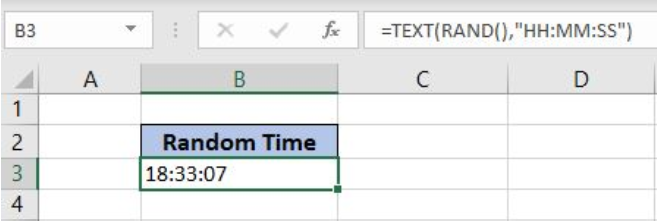Get instant live expert help with Excel or Google Sheets“My Excelchat expert helped me in less than 20 minutes, saving me what would have been 5 hours of work!”

#### Post your problem and you’ll get expert help in seconds.

Your message must be at least 40 characters
Our professional experts are available now. Your privacy is guaranteed.

# Here is How to Create a Random Time Generator in Excel

Excel allows a user to get a random time using the TEXT and RAND functions. This step by step tutorial will assist all levels of Excel users in creating a random time generator.

##Figure 1. The final result of the formula

## Syntax of the RAND Function

The generic formula is:

`=RAND()`

The function has no parameters and returns a random number between 0 and 1.

## Syntax of the TEXT formula

`=TEXT(value, format_text)`

The parameters of the TEXT function are:

• value – a value which we want to format
• format_text – formatting which we want to apply on the value.

## Get a Random Time

We want to get a random decimal number between 0 and 1

The formula looks like:

`=TEXT(RAND(), "HH:MM:SS")`

The value parameter of the TEXT function is a random number as a result of the RAND function. The value parameter is “HH:MM:SS” which is the time format.

To apply the formula, we need to follow these steps:

• Select cell B3 and click on it
• Insert the formula: `=TEXT(RAND(), "HH:MM:SS")`
• Press enter

##Figure 2. Get a random time

Every time we press enter in formula cell, we will get a new time value in B3.

Most of the time, the problem you will need to solve will be more complex than a simple application of a formula or function. If you want to save hours of research and frustration, try our live Excelchat service! Our Excel Experts are available 24/7 to answer any Excel question you may have. We guarantee a connection within 30 seconds and a customized solution within 20 minutes.

Solution examplesGood morning, I need a cell formatting solution to display leading zero in my excel table with the following conditions. 1. Cannot format as text 2. cell size is not fixed. Please let me know if there is a solution.Thanks in advance
Solved by V. C. in 11 minslooking for an Iferror formula that will bring back a blank value if a date is not listed. Currently using the following but want to add an Iferror statement: =TEXT(H2,"DDDD"). H2 is the raw date ex. 2/8/18. I tried =IFERROR(TEXT(H2,"DDDD"),"") but it keeps returning "saturday" instead of a blank cell. Any help is appreciated.
Solved by F. Q. in 20 minsHow do I make this formula =Value(IFerror(TEXT(AM3,"HMM"),"0")) Return a value of 2400 if the value is not an error?
Solved by F. J. in 21 minsI have an excel spreadsheet with two worksheets. And the following formula is not pulling the data. =VLOOKUP(\$A\$2:\$A\$566,'Module Type Info'!\$A\$2:\$D\$97,4,FALSE) In sheet 1, I am using all data in column 1 for the lookup In sheet 2, I have selected the first 4 columns of data for array I want to pull data from sheet 2, column 4 into sheet 1 Both tabs are sorted alphabetically. Confirmed that the value in column 4 of sheet 2 is a TEXT field. Why is this not pulling the info from sheet 2 into sheet 1
Solved by F. L. in 39 minsI'm Apply a =RANDBETWEEN formula in one cells want to apply Formula to entire column and run formula when i hit the entire on the particular cell
Solved by M. S. in 20 mins